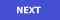What's the distinction between a dominant 7th on C and a dominant 7th in C? Question: What is the distinction between being asked to play the dominant 7th ON C and being asked to play a dominat 7th in the key of C? -L.G.Answer: If the request is for a dominant seventh on C, that would be a dominant seventh chord whose root is C, i.e. C-E-G-Bb: a major triad with an added minor seventh.But if the request is for a dominant seventh "for the key of C" ("in" is not quite as clear), then you'd want the dominant seventh chord that belongs to the key of C, that is, a dominant seventh built on G, which is the dominant degree of C.Every major key has exactly one dominant seventh chord, if you use only the notes of the key. And true to its name, it is the chord that is arises naturally if you make a seventh chord on the fifth, or dominant, note of the scale. So that would be G-B-D-F for the key of C.The seventh chord *on* C, C-E-G-Bb, really belongs to the key of F, where it would be the dominant seventh chord. In the key of C it would be called "V7 of IV," a kind of secondary dominant. Return to Q&A Index